## Digital Frequency Transformations

### Details and Methodology

#### Overview of Transformations

Converting existing FIR or IIR filter designs to a modified IIR form is often done using allpass frequency transformations. Although the resulting designs can be considerably more expensive in terms of dimensionality than the prototype (original) filter, their ease of use in fixed or variable applications is a big advantage.

The general idea of the frequency transformation is to take an existing prototype filter and produce another filter from it that retains some of the characteristics of the prototype, in the frequency domain. Transformation functions achieve this by replacing each delaying element of the prototype filter with an allpass filter carefully designed to have a prescribed phase characteristic for achieving the modifications requested by the designer.

The basic form of mapping commonly used is

`${H}_{T}\left(z\right)={H}_{o}\left[{H}_{A}\left(z\right)\right]$`

The HA(z) is an Nth-order allpass mapping filter given by

`$\begin{array}{l}{H}_{A}\left(z\right)=S\frac{\sum _{i=0}^{N}{\alpha }_{i}{z}^{-i}}{\sum _{i=0}^{N}{\alpha }_{i}{z}^{-N+i}}=\frac{{N}_{A}\left(z\right)}{{D}_{A}\left(z\right)}\\ {\alpha }_{0}=1\end{array}$`

where

Ho(z) — Transfer function of the prototype filter

HA(z) — Transfer function of the allpass mapping filter

HT(z) — Transfer function of the target filter

Let's look at a simple example of the transformation given by

`${H}_{T}\left(z\right)={H}_{o}\left(-z\right)$`

The target filter has its poles and zeroes flipped across the origin of the real and imaginary axes. For the real filter prototype, it gives a mirror effect against 0.5, which means that lowpass Ho(z) gives rise to a real highpass HT(z). This is shown in the following figure for the prototype filter designed as a third-order halfband elliptic filter.

Example of a Simple Mirror Transformation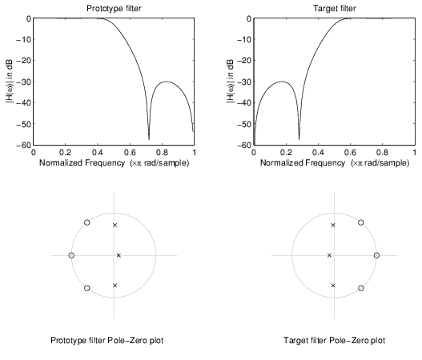The choice of an allpass filter to provide the frequency mapping is necessary to provide the frequency translation of the prototype filter frequency response to the target filter by changing the frequency position of the features from the prototype filter without affecting the overall shape of the filter response.

The phase response of the mapping filter normalized to π can be interpreted as a translation function:

`$H\left({w}_{new}\right)={\omega }_{old}$`

The graphical interpretation of the frequency transformation is shown in the figure below. The complex multiband transformation takes a real lowpass filter and converts it into a number of passbands around the unit circle.

Graphical Interpretation of the Mapping Process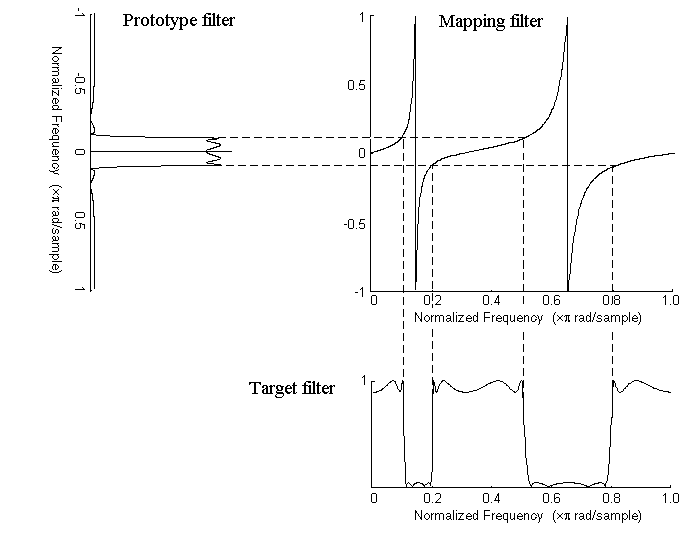Most of the frequency transformations are based on the second-order allpass mapping filter:

`${H}_{A}\left(z\right)=±\frac{1+{\alpha }_{1}{z}^{-1}+{\alpha }_{2}{z}^{-2}}{{\alpha }_{2}+{\alpha }_{1}{z}^{-1}+{z}^{-2}}$`

The two degrees of freedom provided by α1 and α2 choices are not fully used by the usual restrictive set of “flat-top” classical mappings like lowpass to bandpass. Instead, any two transfer function features can be migrated to (almost) any two other frequency locations if α1 and α2 are chosen so as to keep the poles of HA(z) strictly outside the unit circle (since HA(z) is substituted for z in the prototype transfer function). Moreover, as first pointed out by Constantinides, the selection of the outside sign influences whether the original feature at zero can be moved (the minus sign, a condition known as “DC mobility”) or whether the Nyquist frequency can be migrated (the “Nyquist mobility” case arising when the leading sign is positive).

#### Select Features Subject to Transformation

Choosing the appropriate frequency transformation for achieving the required effect and the correct features of the prototype filter is very important and needs careful consideration. It is not advisable to use a first-order transformation for controlling more than one feature. The mapping filter will not give enough flexibility. It is also not good to use higher order transformation just to change the cutoff frequency of the lowpass filter. The increase of the filter order would be too big, without considering the additional replica of the prototype filter that may be created in undesired places.

Feature Selection for Real Lowpass to Bandpass Transformation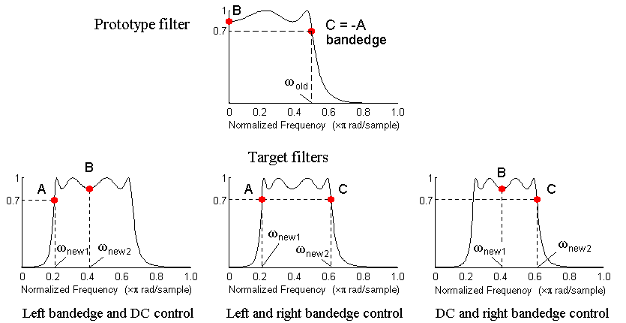To illustrate the idea, the second-order real multipoint transformation was applied three times to the same elliptic halfband filter in order to make it into a bandpass filter. In each of the three cases, two different features of the prototype filter were selected in order to obtain a bandpass filter with passband ranging from 0.25 to 0.75. The position of the DC feature was not important, but it would be advantageous if it were in the middle between the edges of the passband in the target filter. In the first case the selected features were the left and the right band edges of the lowpass filter passband, in the second case they were the left band edge and the DC, in the third case they were DC and the right band edge.

Result of Choosing Different Features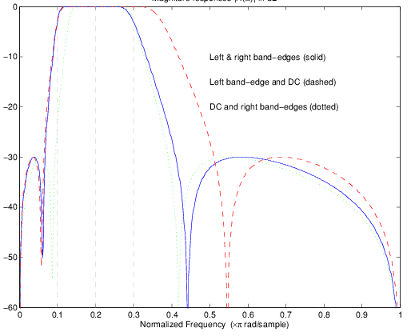The results of all three approaches are completely different. For each of them only the selected features were positioned precisely where they were required. In the first case the DC is moved toward the left passband edge just like all the other features close to the left edge being squeezed there. In the second case the right passband edge was pushed way out of the expected target as the precise position of DC was required. In the third case the left passband edge was pulled toward the DC in order to position it at the correct frequency. The conclusion is that if only the DC can be anywhere in the passband, the edges of the passband should have been selected for the transformation. For most of the cases requiring the positioning of passbands and stopbands, designers should always choose the position of the edges of the prototype filter in order to make sure that they get the edges of the target filter in the correct places. Frequency responses for the three cases considered are shown in the figure. The prototype filter was a third-order elliptic lowpass filter with cutoff frequency at 0.5.

The MATLAB® code used to generate the figure is given here.

The prototype filter is a halfband elliptic, real, third-order lowpass filter:

`[b, a] = ellip(3, 0.1, 30, 0.409);`

In the example the requirements are set to create a real bandpass filter with passband edges at 0.1 and 0.3 out of the real lowpass filter having the cutoff frequency at 0.5. This is attempted in three different ways. In the first approach both edges of the passband are selected, in the second approach the left edge of the passband and the DC are chosen, while in the third approach the DC and the right edge of the passband are taken:

```[num1,den1] = iirlp2xn(b, a, [-0.5, 0.5], [0.1, 0.3]); [num2,den2] = iirlp2xn(b, a, [-0.5, 0.0], [0.1, 0.2]); [num3,den3] = iirlp2xn(b, a, [ 0.0, 0.5], [0.2, 0.3]);```

#### Mapping from Prototype Filter to Target Filter

In general the frequency mapping converts the prototype filter, Ho(z), to the target filter, HT(z), using the NAth-order allpass filter, HA(z). The general form of the allpass mapping filter is given in Overview of Transformations. The frequency mapping is a mathematical operation that replaces each delayer of the prototype filter with an allpass filter. There are two ways of performing such mapping. The choice of the approach is dependent on how prototype and target filters are represented.

When the Nth-order prototype filter is given with pole-zero form

`${H}_{o}\left(z\right)=\frac{\sum _{i=1}^{N}\left(z-{z}_{i}\right)}{\sum _{i=1}^{N}\left(z-{p}_{i}\right)}$`

the mapping will replace each pole, pi, and each zero, zi, with a number of poles and zeros equal to the order of the allpass mapping filter:

`${H}_{o}\left(z\right)=\frac{\sum _{i=1}^{N}\left(S\sum _{k=0}^{N-1}{\alpha }_{k}{z}^{k}-{z}_{i}\cdot \sum _{k=0}^{N-1}{\alpha }_{k}{z}^{N}{}^{-k}\right)}{\sum _{i=1}^{N}\left(S\sum _{k=0}^{N-1}{\alpha }_{k}{z}^{k}-{p}_{i}\cdot \sum _{k=0}^{N-1}{\alpha }_{k}{z}^{N}{}^{-k}\right)}$`
``

The root finding needs to be used on the bracketed expressions in order to find the poles and zeros of the target filter.

When the prototype filter is described in the numerator-denominator form:

`${H}_{T}\left(z\right)={\frac{{\beta }_{0}{z}^{N}+{\beta }_{1}{z}^{N-1}+\dots +{\beta }_{N}}{{\alpha }_{0}{z}^{N}+{\alpha }_{1}{z}^{N-1}+\dots +{\alpha }_{N}}|}_{z={H}_{A}\left(z\right)}$`

Then the mapping process will require a number of convolutions in order to calculate the numerator and denominator of the target filter:

`${H}_{T}\left(z\right)=\frac{{\beta }_{1}{N}_{A}{\left(z\right)}^{N}+{\beta }_{2}{N}_{A}{\left(z\right)}^{N-1}{D}_{A}\left(z\right)+\dots +{\beta }_{N}{D}_{A}{\left(z\right)}^{N}}{{\beta }_{1}{N}_{A}{\left(z\right)}^{N}+{\beta }_{2}{N}_{A}{\left(z\right)}^{N-1}{D}_{A}\left(z\right)+\dots +{\beta }_{N}{D}_{A}{\left(z\right)}^{N}}$`

For each coefficient αi and βi of the prototype filter the NAth-order polynomials must be convolved `N` times. Such approach may cause rounding errors for large prototype filters and/or high order mapping filters. In such a case the user should consider the alternative of doing the mapping using via poles and zeros.

#### Summary of Frequency Transformations

• Most frequency transformations are described by closed-form solutions or can be calculated from the set of linear equations.

• They give predictable and familiar results.

• Ripple heights from the prototype filter are preserved in the target filter.

• They are architecturally appealing for variable and adaptive filters.

• There are cases when using optimization methods to design the required filter gives better results.

• High-order transformations increase the dimensionality of the target filter, which may give expensive final results.

• Starting from fresh designs helps avoid locked-in compromises.

### Frequency Transformations for Real Filters

#### Overview

This section discusses real frequency transformations that take the real lowpass prototype filter and convert it into a different real target filter. The target filter has its frequency response modified in respect to the frequency response of the prototype filter according to the characteristic of the applied frequency transformation.

#### Real Frequency Shift

Real frequency shift transformation uses a second-order allpass mapping filter. It performs an exact mapping of one selected feature of the frequency response into its new location, additionally moving both the Nyquist and DC features. This effectively moves the whole response of the lowpass filter by the distance specified by the selection of the feature from the prototype filter and the target filter. As a real transformation, it works in a similar way for positive and negative frequencies.

`${H}_{A}\left(z\right)={z}^{-1}\cdot \frac{1-\alpha {z}^{-1}}{\alpha -{z}^{-1}}$`

with α given by

`$\alpha =\left\{\begin{array}{lll}\frac{\mathrm{cos}\frac{\pi }{2}\left({\omega }_{old}-2{\omega }_{new}\right)}{\mathrm{cos}\frac{\pi }{2}{\omega }_{old}}\hfill & for\hfill & |\mathrm{cos}\frac{\pi }{2}\left({\omega }_{old}-2{\omega }_{new}\right)|<1\hfill \\ \frac{\mathrm{sin}\frac{\pi }{2}\left({\omega }_{old}-2{\omega }_{new}\right)}{\mathrm{sin}\frac{\pi }{2}{\omega }_{old}}\hfill & otherwise\hfill & \hfill \end{array}$`

where

ωold — Frequency location of the selected feature in the prototype filter

ωnew — Position of the feature originally at ωold in the target filter

The following example shows how this transformation can be used to move the response of the prototype lowpass filter in either direction. Please note that because the target filter must also be real, the response of the target filter will inherently be disturbed at frequencies close to Nyquist and close to DC. Here is the MATLAB code for generating the example in the figure.

The prototype filter is a halfband elliptic, real, third-order lowpass filter:

`[b, a] = ellip(3, 0.1, 30, 0.409);`

The example transformation moves the feature originally at 0.5 to 0.9:

`[num,den] = iirshift(b, a, 0.5, 0.9);`

Example of Real Frequency Shift Mapping#### Real Lowpass to Real Lowpass

Real lowpass filter to real lowpass filter transformation uses a first-order allpass mapping filter. It performs an exact mapping of one feature of the frequency response into the new location keeping DC and Nyquist features fixed. As a real transformation, it works in a similar way for positive and negative frequencies. It is important to mention that using first-order mapping ensures that the order of the filter after the transformation is the same as it was originally.

`${H}_{A}\left(z\right)=-\left(\frac{1-\alpha {z}^{-1}}{\alpha -{z}^{-1}}\right)$`

with α given by

`$\alpha =\frac{\mathrm{sin}\frac{\pi }{2}\left({\omega }_{old}-{\omega }_{new}\right)}{\mathrm{sin}\frac{\pi }{2}\left({\omega }_{old}-{\omega }_{new}\right)}$`

where

ωold — Frequency location of the selected feature in the prototype filter

ωnew — Frequency location of the same feature in the target filter

The example below shows how to modify the cutoff frequency of the prototype filter. The MATLAB code for this example is shown in the following figure.

The prototype filter is a halfband elliptic, real, third-order filter:

`[b, a] = ellip(3, 0.1, 30, 0.409);`

The cutoff frequency moves from 0.5 to 0.75:

`[num,den] = iirlp2lp(b, a, 0.5, 0.75);`

Example of Real Lowpass to Real Lowpass Mapping#### Real Lowpass to Real Highpass

Real lowpass filter to real highpass filter transformation uses a first-order allpass mapping filter. It performs an exact mapping of one feature of the frequency response into the new location additionally swapping DC and Nyquist features. As a real transformation, it works in a similar way for positive and negative frequencies. Just like in the previous transformation because of using a first-order mapping, the order of the filter before and after the transformation is the same.

`${H}_{A}\left(z\right)=-\left(\frac{1+\alpha {z}^{-1}}{\alpha +{z}^{-1}}\right)$`

with α given by

`$\alpha =-\left(\frac{\mathrm{cos}\frac{\pi }{2}\left({\omega }_{old}+{\omega }_{new}\right)}{\mathrm{cos}\frac{\pi }{2}\left({\omega }_{old}-{\omega }_{new}\right)}\right)$`

where

ωold — Frequency location of the selected feature in the prototype filter

ωnew — Frequency location of the same feature in the target filter

The example below shows how to convert the lowpass filter into a highpass filter with arbitrarily chosen cutoff frequency. In the MATLAB code below, the lowpass filter is converted into a highpass with cutoff frequency shifted from 0.5 to 0.75. Results are shown in the figure.

The prototype filter is a halfband elliptic, real, third-order filter:

`[b, a] = ellip(3, 0.1, 30, 0.409);`

The example moves the cutoff frequency from 0.5 to 0.75:

```[num,den] = iirlp2hp(b, a, 0.5, 0.75); ```

Example of Real Lowpass to Real Highpass Mapping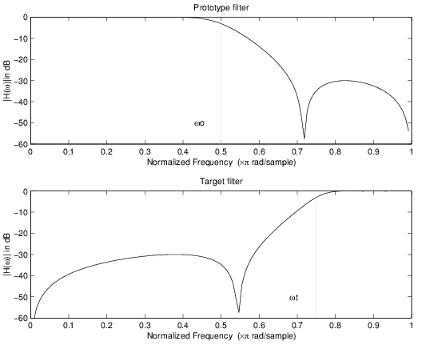#### Real Lowpass to Real Bandpass

Real lowpass filter to real bandpass filter transformation uses a second-order allpass mapping filter. It performs an exact mapping of two features of the frequency response into their new location additionally moving a DC feature and keeping the Nyquist feature fixed. As a real transformation, it works in a similar way for positive and negative frequencies.

`${H}_{A}\left(z\right)=-\left(\frac{1-\beta \left(1+\alpha \right){z}^{-1}-\alpha {z}^{-2}}{\alpha -\beta \left(1+\alpha \right){z}^{-1}+{z}^{-2}}\right)$`

with α and β given by

`$\alpha =\frac{\mathrm{sin}\frac{\pi }{4}\left(2{\omega }_{old}-{\omega }_{new,2}+{\omega }_{new,1}\right)}{\mathrm{sin}\frac{\pi }{4}\left(2{\omega }_{old}+{\omega }_{new,2}-{\omega }_{new,1}\right)}$`
`$\beta =\mathrm{cos}\frac{\pi }{2}\left({\omega }_{new,1}+{\omega }_{new,2}\right)$`

where

ωold — Frequency location of the selected feature in the prototype filter

ωnew,1 — Position of the feature originally at (-ωold) in the target filter

ωnew,2 — Position of the feature originally at (+ωold) in the target filter

The example below shows how to move the response of the prototype lowpass filter in either direction. Please note that because the target filter must also be real, the response of the target filter will inherently be disturbed at frequencies close to Nyquist and close to DC. Here is the MATLAB code for generating the example in the figure.

The prototype filter is a halfband elliptic, real, third-order lowpass filter:

`[b, a] = ellip(3, 0.1, 30, 0.409);`

The example transformation creates the passband between 0.5 and 0.75:

`[num,den] = iirlp2bp(b, a, 0.5, [0.5, 0.75]);`

Example of Real Lowpass to Real Bandpass Mapping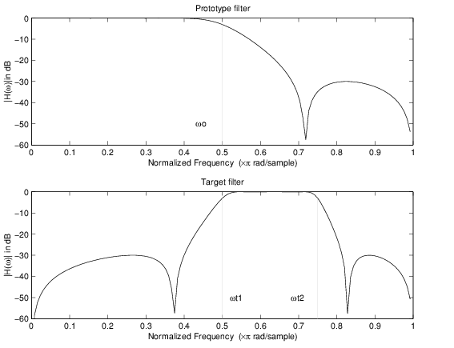#### Real Lowpass to Real Bandstop

Real lowpass filter to real bandstop filter transformation uses a second-order allpass mapping filter. It performs an exact mapping of two features of the frequency response into their new location additionally moving a Nyquist feature and keeping the DC feature fixed. This effectively creates a stopband between the selected frequency locations in the target filter. As a real transformation, it works in a similar way for positive and negative frequencies.

`${H}_{A}\left(z\right)=\frac{1-\beta \left(1+\alpha \right){z}^{-1}+\alpha {z}^{-2}}{\alpha -\beta \left(1+\alpha \right){z}^{-1}+{z}^{-2}}$`

with α and β given by

`$\begin{array}{l}\alpha =\frac{\mathrm{cos}\frac{\pi }{4}\left(2{\omega }_{old}+{\omega }_{new,2}-{\omega }_{new,1}\right)}{\mathrm{cos}\frac{\pi }{4}\left(2{\omega }_{old}-{\omega }_{new,2}+{\omega }_{new,1}\right)}\\ \beta =\mathrm{cos}\frac{\pi }{2}\left({\omega }_{new,1}+{\omega }_{new,2}\right)\end{array}$`

where

ωold — Frequency location of the selected feature in the prototype filter

ωnew,1 — Position of the feature originally at (-ωold) in the target filter

ωnew,2 — Position of the feature originally at (+ωold) in the target filter

The following example shows how this transformation can be used to convert the prototype lowpass filter with cutoff frequency at 0.5 into a real bandstop filter with the same passband and stopband ripple structure and stopband positioned between 0.5 and 0.75. Here is the MATLAB code for generating the example in the figure.

The prototype filter is a halfband elliptic, real, third-order lowpass filter:

`[b, a] = ellip(3, 0.1, 30, 0.409);`

The example transformation creates a stopband from 0.5 to 0.75:

`[num,den] = iirlp2bs(b, a, 0.5, [0.5, 0.75]);`

Example of Real Lowpass to Real Bandstop Mapping#### Real Lowpass to Real Multiband

This high-order transformation performs an exact mapping of one selected feature of the prototype filter frequency response into a number of new locations in the target filter. Its most common use is to convert a real lowpass with predefined passband and stopband ripples into a real multiband filter with N arbitrary band edges, where N is the order of the allpass mapping filter.

`$\begin{array}{l}{H}_{A}\left(z\right)=S\frac{\sum _{i=0}^{N}{\alpha }_{i}^{}{z}^{-i}}{\sum _{i=0}^{N}{\alpha }_{i}^{}{z}^{-N+i}}\\ {\alpha }_{0}=1\end{array}$`

The coefficients α are given by

`$\left\{\begin{array}{l}\begin{array}{cc}{\alpha }_{0}=1& k=1,\dots ,N\end{array}\\ {\alpha }_{k}=-S\frac{\mathrm{sin}\frac{\pi }{2}\left(N{\omega }_{new}+{\left(-1\right)}^{k}{\omega }_{old}\right)}{\mathrm{sin}\frac{\pi }{2}\left(\left(N-2k\right){\omega }_{new}+{\left(-1\right)}^{k}{\omega }_{old}\right)}\end{array}$`

where

ωold,k — Frequency location of the first feature in the prototype filter

ωnew,k — Position of the feature originally at ωold,k in the target filter

The mobility factor, S, specifies the mobility or either DC or Nyquist feature:

`$S=\left\{\begin{array}{cc}1& Nyquist\\ -1& DC\end{array}$`

The example below shows how this transformation can be used to convert the prototype lowpass filter with cutoff frequency at 0.5 into a filter having a number of bands positioned at arbitrary edge frequencies 1/5, 2/5, 3/5 and 4/5. Parameter `S` was such that there is a passband at DC. Here is the MATLAB code for generating the figure.

The prototype filter is a halfband elliptic, real, third-order lowpass filter:

`[b, a] = ellip(3, 0.1, 30, 0.409);`

The example transformation creates three stopbands, from DC to 0.2, from 0.4 to 0.6 and from 0.8 to Nyquist:

`[num,den] = iirlp2mb(b, a, 0.5, [0.2, 0.4, 0.6, 0.8], `pass');`

Example of Real Lowpass to Real Multiband Mapping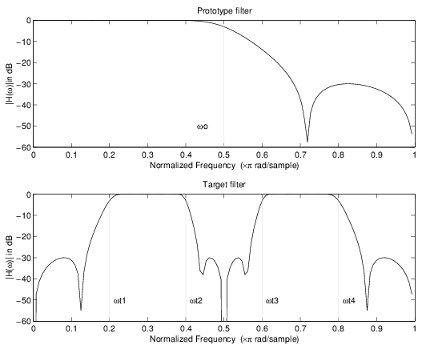#### Real Lowpass to Real Multipoint

This high-order frequency transformation performs an exact mapping of a number of selected features of the prototype filter frequency response to their new locations in the target filter. The mapping filter is given by the general IIR polynomial form of the transfer function as given below.

`$\begin{array}{l}{H}_{A}\left(z\right)=S\frac{\sum _{i=0}^{N}{\alpha }_{i}{z}^{-i}}{\sum _{i=0}^{N}{\alpha }_{i}{z}^{-N+i}}\\ {\alpha }_{0}=1\end{array}$`

For the Nth-order multipoint frequency transformation the coefficients α are

`$\left\{\begin{array}{l}\sum _{i=1}^{N}{\alpha }_{N-i}{z}_{old,k}\cdot {z}_{new,k}^{i}-S\cdot {z}_{new,k}^{N-i}=-{z}_{old,k}-S\cdot {z}_{new,k}\hfill \\ {z}_{old,k}={e}^{j\pi {\omega }_{old,k}}\hfill \\ {z}_{new,k}={e}^{j\pi {\omega }_{new,k}}\hfill \\ k=1,\dots ,N\hfill \end{array}$`

where

ωold,k — Frequency location of the first feature in the prototype filter

ωnew,k — Position of the feature originally at ωold,k in the target filter

The mobility factor, S, specifies the mobility of either DC or Nyquist feature:

`$S=\left\{\begin{array}{cc}1& Nyquist\\ -1& DC\end{array}$`

The example below shows how this transformation can be used to move features of the prototype lowpass filter originally at -0.5 and 0.5 to their new locations at 0.5 and 0.75, effectively changing a position of the filter passband. Here is the MATLAB code for generating the figure.

The prototype filter is a halfband elliptic, real, third-order lowpass filter:

`[b, a] = ellip(3, 0.1, 30, 0.409);`

The example transformation creates a passband from 0.5 to 0.75:

`[num,den] = iirlp2xn(b, a, [-0.5, 0.5], [0.5, 0.75], `pass');`

Example of Real Lowpass to Real Multipoint Mapping### Frequency Transformations for Complex Filters

#### Overview

This section discusses complex frequency transformation that take the complex prototype filter and convert it into a different complex target filter. The target filter has its frequency response modified in respect to the frequency response of the prototype filter according to the characteristic of the applied frequency transformation from:

#### Complex Frequency Shift

Complex frequency shift transformation is the simplest first-order transformation that performs an exact mapping of one selected feature of the frequency response into its new location. At the same time it rotates the whole response of the prototype lowpass filter by the distance specified by the selection of the feature from the prototype filter and the target filter.

`${H}_{A}\left(z\right)=\alpha {z}^{-1}$`

with α given by

`$\alpha ={e}^{j2\pi \left({\nu }_{new}-{\nu }_{old}\right)}$`

where

ωold — Frequency location of the selected feature in the prototype filter

ωnew — Position of the feature originally at ωold in the target filter

A special case of the complex frequency shift is a, so called, Hilbert Transformer. It can be designed by setting the parameter to |α|=1, that is

`$\alpha =\left\{\begin{array}{cc}1& forward\\ -1& inverse\end{array}$`

The example below shows how to apply this transformation to rotate the response of the prototype lowpass filter in either direction. Please note that because the transformation can be achieved by a simple phase shift operator, all features of the prototype filter will be moved by the same amount. Here is the MATLAB code for generating the example in the figure.

The prototype filter is a halfband elliptic, real, third-order lowpass filter:

`[b, a] = ellip(3, 0.1, 30, 0.409);`

The example transformation moves the feature originally at 0.5 to 0.3:

`[num,den] = iirshiftc(b, a, 0.5, 0.3);`

Example of Complex Frequency Shift Mapping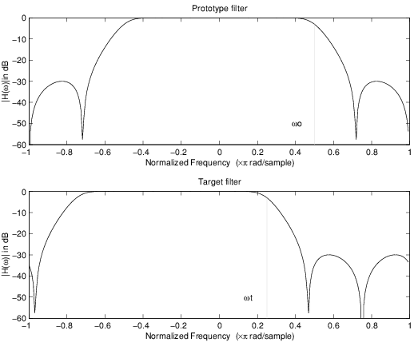#### Real Lowpass to Complex Bandpass

This first-order transformation performs an exact mapping of one selected feature of the prototype filter frequency response into two new locations in the target filter creating a passband between them. Both Nyquist and DC features can be moved with the rest of the frequency response.

`${H}_{A}\left(z\right)=\frac{\beta -\alpha {z}^{-1}}{{z}^{-1}-\alpha \beta }$`

with α and β are given by

`$\begin{array}{l}\alpha =\frac{\mathrm{sin}\frac{\pi }{4}\left(2{\omega }_{old}-{\omega }_{new,2}+{\omega }_{new,1}\right)}{\mathrm{sin}\pi \left(2{\omega }_{old}+{\omega }_{new,2}-{\omega }_{new,1}\right)}\\ \beta ={e}^{-j\pi \left({\omega }_{new}-{\omega }_{old}\right)}\end{array}$`

where

ωold — Frequency location of the selected feature in the prototype filter

ωnew,1 — Position of the feature originally at (-ωold) in the target filter

ωnew,2 — Position of the feature originally at (+ωold) in the target filter

The following example shows the use of such a transformation for converting a real halfband lowpass filter into a complex bandpass filter with band edges at 0.5 and 0.75. Here is the MATLAB code for generating the example in the figure.

The prototype filter is a half band elliptic, real, third-order lowpass filter:

`[b, a] = ellip(3, 0.1, 30, 0.409);`

The transformation creates a passband from 0.5 to 0.75:

`[num,den] = iirlp2bpc(b, a, 0.5, [0.5 0.75]);`

Example of Real Lowpass to Complex Bandpass Mapping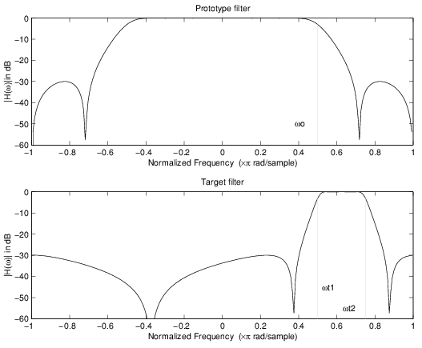#### Real Lowpass to Complex Bandstop

This first-order transformation performs an exact mapping of one selected feature of the prototype filter frequency response into two new locations in the target filter creating a stopband between them. Both Nyquist and DC features can be moved with the rest of the frequency response.

`${H}_{A}\left(z\right)=\frac{\beta -\alpha {z}^{-1}}{\alpha \beta -{z}^{-1}}$`

with α and β are given by

`$\begin{array}{l}\alpha =\frac{\mathrm{cos}\pi \left(2{\omega }_{old}+{\nu }_{new,2}-{\nu }_{new,1}\right)}{\mathrm{cos}\pi \left(2{\omega }_{old}-{\nu }_{new,2}+{\nu }_{new,1}\right)}\\ \beta ={e}^{-j\pi \left({\omega }_{new}-{\omega }_{old}\right)}\end{array}$`

where

ωold — Frequency location of the selected feature in the prototype filter

ωnew,1 — Position of the feature originally at (-ωold) in the target filter

ωnew,2 — Position of the feature originally at (+ωold) in the target filter

The example below shows the use of such a transformation for converting a real halfband lowpass filter into a complex bandstop filter with band edges at 0.5 and 0.75. Here is the MATLAB code for generating the example in the figure.

The prototype filter is a halfband elliptic, real, third-order lowpass filter:

`[b, a] = ellip(3, 0.1, 30, 0.409);`

The transformation creates a stopband from 0.5 to 0.75:

`[num,den] = iirlp2bsc(b, a, 0.5, [0.5 0.75]);`

Example of Real Lowpass to Complex Bandstop Mapping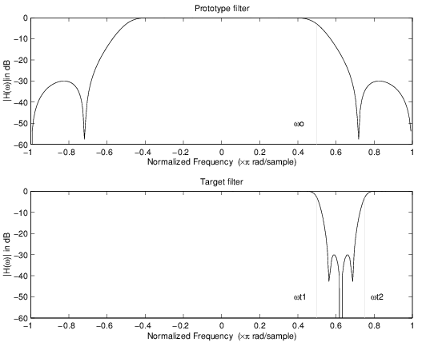#### Real Lowpass to Complex Multiband

This high-order transformation performs an exact mapping of one selected feature of the prototype filter frequency response into a number of new locations in the target filter. Its most common use is to convert a real lowpass with predefined passband and stopband ripples into a multiband filter with arbitrary band edges. The order of the mapping filter must be even, which corresponds to an even number of band edges in the target filter. The Nth-order complex allpass mapping filter is given by the following general transfer function form:

`$\begin{array}{l}{H}_{A}\left(z\right)=S\frac{\sum _{i=0}^{N}{\alpha }_{i}{z}^{-i}}{\sum _{i=0}^{N}{\alpha }_{i}±{z}^{-N+i}}\\ {\alpha }_{0}=1\end{array}$`

The coefficients α are calculated from the system of linear equations:

`$\left\{\begin{array}{l}\sum _{i=1}^{N}\Re \left({\alpha }_{i}\right)\cdot \left[\mathrm{cos}{\beta }_{1,k}-\mathrm{cos}{\beta }_{2,k}\right]+\Im \left({\alpha }_{i}\right)\cdot \left[\mathrm{sin}{\beta }_{1,k}+\mathrm{sin}{\beta }_{2,k}\right]=\mathrm{cos}{\beta }_{3,k}\\ \sum _{i=1}^{N}\Re \left({\alpha }_{i}\right)\cdot \left[\mathrm{sin}{\beta }_{1,k}-\mathrm{sin}{\beta }_{2,k}\right]-\Im \left({\alpha }_{i}\right)\cdot \left[\mathrm{cos}{\beta }_{1,k}+\mathrm{cos}{\beta }_{2,k}\right]=\mathrm{sin}{\beta }_{3,k}\\ {\beta }_{1,k}=-\pi \left[{\nu }_{old}\cdot {\left(-1\right)}^{k}+{\nu }_{new,k}\left(N-k\right)\right]\\ {\beta }_{2,k}=-\pi \left[\Delta C+{\nu }_{new,k}k\right]\\ {\beta }_{3,k}=-\pi \left[{\nu }_{old}\cdot {\left(-1\right)}^{k}+{\nu }_{new,k}N\right]\\ k=1\dots N\end{array}$`

where

ωold — Frequency location of the selected feature in the prototype filter

ωnew,i — Position of features originally at ±ωold in the target filter

Parameter S is the additional rotation factor by the frequency distance ΔC, giving the additional flexibility of achieving the required mapping:

`$S={e}^{-j\pi \Delta C}$`

The example shows the use of such a transformation for converting a prototype real lowpass filter with the cutoff frequency at 0.5 into a multiband complex filter with band edges at 0.2, 0.4, 0.6 and 0.8, creating two passbands around the unit circle. Here is the MATLAB code for generating the figure.

Example of Real Lowpass to Complex Multiband MappingThe prototype filter is a halfband elliptic, real, third-order lowpass filter:

`[b, a] = ellip(3, 0.1, 30, 0.409);`

The example transformation creates two complex passbands:

`[num,den] = iirlp2mbc(b, a, 0.5, [0.2, 0.4, 0.6, 0.8]);`

#### Real Lowpass to Complex Multipoint

This high-order transformation performs an exact mapping of a number of selected features of the prototype filter frequency response to their new locations in the target filter. The Nth-order complex allpass mapping filter is given by the following general transfer function form.

`$\begin{array}{l}{H}_{A}\left(z\right)=S\frac{\sum _{i=0}^{N}{\alpha }_{i}{z}^{-i}}{\sum _{i=0}^{N}{\alpha }_{i}±{z}^{-N+i}}\\ {\alpha }_{0}=1\end{array}$`

The coefficients α can be calculated from the system of linear equations:

`$\left\{\begin{array}{l}\sum _{i=1}^{N}\Re \left({\alpha }_{i}\right)\cdot \left[\mathrm{cos}{\beta }_{1,k}-\mathrm{cos}{\beta }_{2,k}\right]+\Im \left({\alpha }_{i}\right)\cdot \left[\mathrm{sin}{\beta }_{1,k}+\mathrm{sin}{\beta }_{2,k}\right]=\mathrm{cos}{\beta }_{3,k}\\ \sum _{i=1}^{N}\Re \left({\alpha }_{i}\right)\cdot \left[\mathrm{sin}{\beta }_{1,k}-\mathrm{sin}{\beta }_{2,k}\right]-\Im \left({\alpha }_{i}\right)\cdot \left[\mathrm{cos}{\beta }_{1,k}+\mathrm{cos}{\beta }_{2,k}\right]=\mathrm{sin}{\beta }_{3,k}\\ {\beta }_{1,k}=-\frac{\pi }{2}\left[{\omega }_{old,k}+{\omega }_{new,k}\left(N-k\right)\right]\\ {\beta }_{2,k}=-\frac{\pi }{2}\left[2\Delta C+{\omega }_{new,k}k\right]\\ {\beta }_{3,k}=-\frac{\pi }{2}\left[{\omega }_{old,k}+{\omega }_{new,k}N\right]\\ k=1\dots N\end{array}$`

where

ωold,k — Frequency location of the first feature in the prototype filter

ωnew,k — Position of the feature originally at ωold,k in the target filter

Parameter S is the additional rotation factor by the frequency distance ΔC, giving the additional flexibility of achieving the required mapping:

`$S={e}^{-j\pi \Delta C}$`

The following example shows how this transformation can be used to move one selected feature of the prototype lowpass filter originally at -0.5 to two new frequencies -0.5 and 0.1, and the second feature of the prototype filter from 0.5 to new locations at -0.25 and 0.3. This creates two nonsymmetric passbands around the unit circle, creating a complex filter. Here is the MATLAB code for generating the figure.

The prototype filter is a halfband elliptic, real, third-order lowpass filter:

`[b, a] = ellip(3, 0.1, 30, 0.409);`

The example transformation creates two nonsymmetric passbands:

`[num,den] = iirlp2xc(b,a,0.5*[-1,1,-1,1], [-0.5,-0.25,0.1,0.3]);`

Example of Real Lowpass to Complex Multipoint Mapping#### Complex Bandpass to Complex Bandpass

This first-order transformation performs an exact mapping of two selected features of the prototype filter frequency response into two new locations in the target filter. Its most common use is to adjust the edges of the complex bandpass filter.

`${H}_{A}\left(z\right)=\frac{\alpha \left(\gamma -\beta {z}^{-1}\right)}{{z}^{-1}-\beta \gamma }$`

with α and β are given by

`$\begin{array}{l}\alpha =\frac{\mathrm{sin}\frac{\pi }{4}\left(\left({\omega }_{old,2}-{\omega }_{old,1}\right)-\left({\omega }_{new,2}-{\omega }_{new,1}\right)\right)}{\mathrm{sin}\frac{\pi }{4}\left(\left({\omega }_{old,2}-{\omega }_{old,1}\right)+\left({\omega }_{new,2}-{\omega }_{new,1}\right)\right)}\\ \alpha ={e}^{-j\pi \left({\omega }_{old,2}-{\omega }_{old,1}\right)}\\ \gamma ={e}^{-j\pi \left({\omega }_{new,2}-{\omega }_{new,1}\right)}\end{array}$`

where

ωold,1 — Frequency location of the first feature in the prototype filter

ωold,2 — Frequency location of the second feature in the prototype filter

ωnew,1 — Position of the feature originally at ωold,1 in the target filter

ωnew,2 — Position of the feature originally at ωold,2 in the target filter

The following example shows how this transformation can be used to modify the position of the passband of the prototype filter, either real or complex. In the example below the prototype filter passband spanned from 0.5 to 0.75. It was converted to having a passband between -0.5 and 0.1. Here is the MATLAB code for generating the figure.

The prototype filter is a halfband elliptic, real, third-order lowpass filter:

`[b, a] = ellip(3, 0.1, 30, 0.409);`

The example transformation creates a passband from 0.25 to 0.75:

`[num,den] = iirbpc2bpc(b, a, [0.25, 0.75], [-0.5, 0.1]);`

Example of Complex Bandpass to Complex Bandpass Mapping